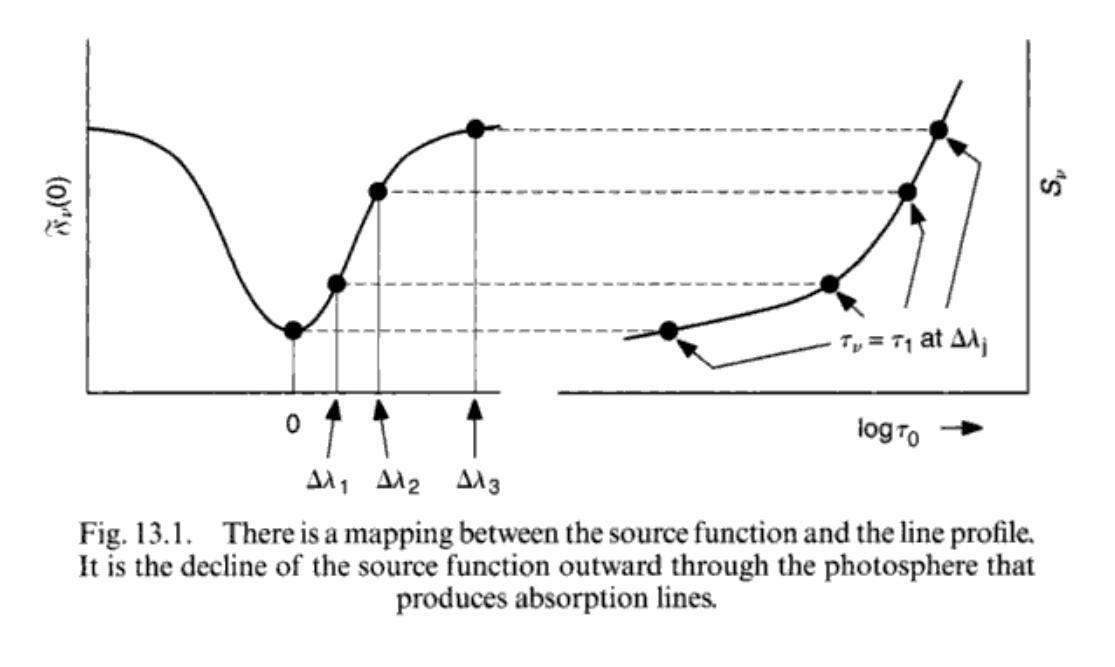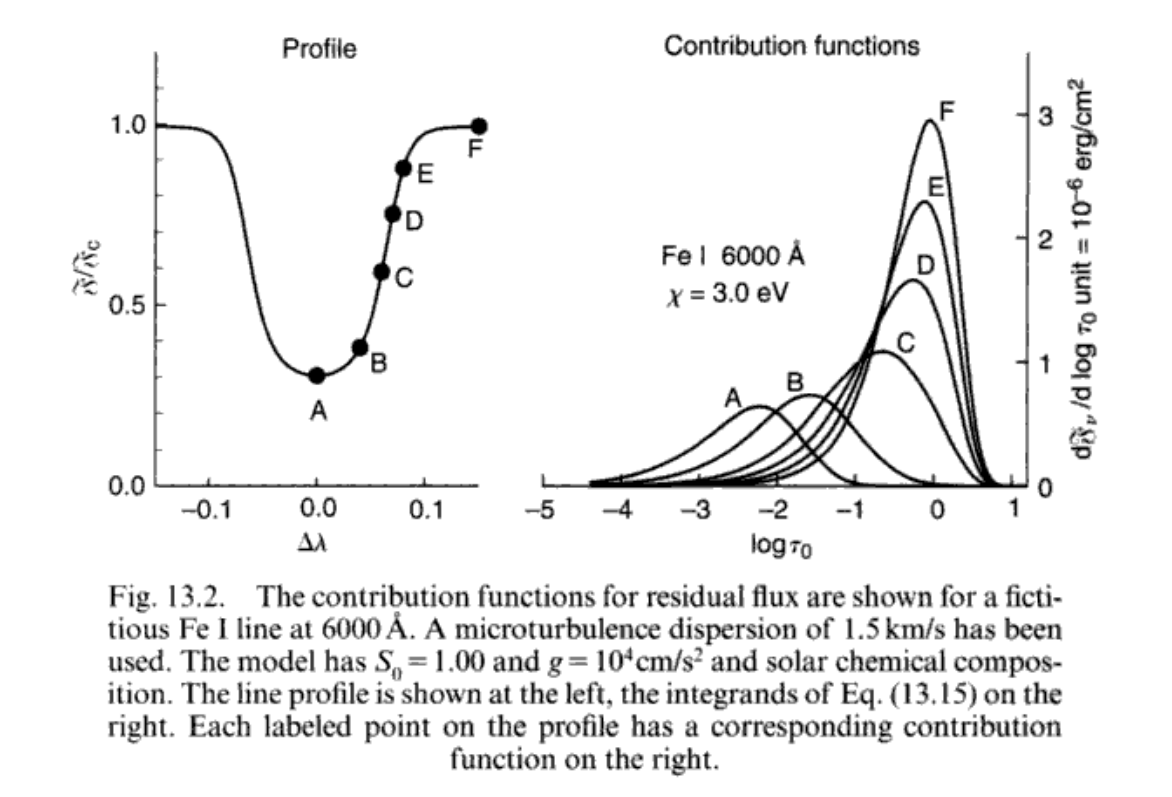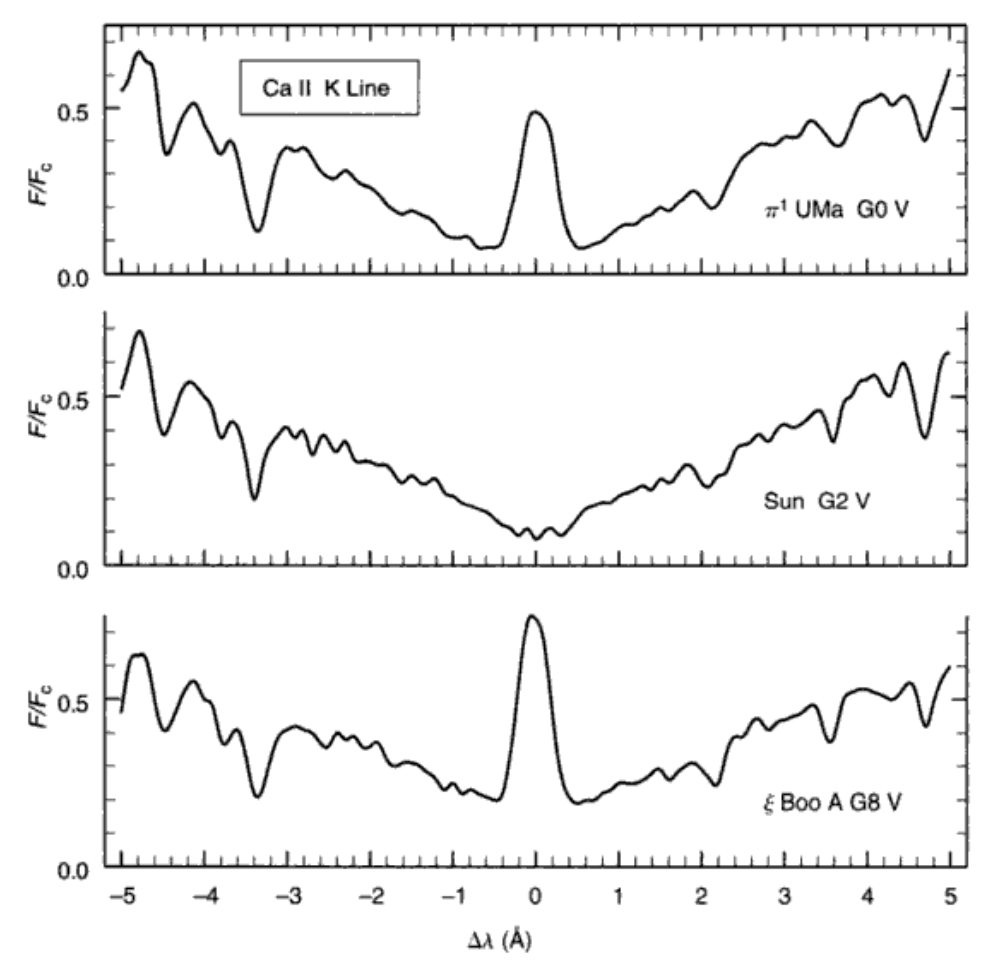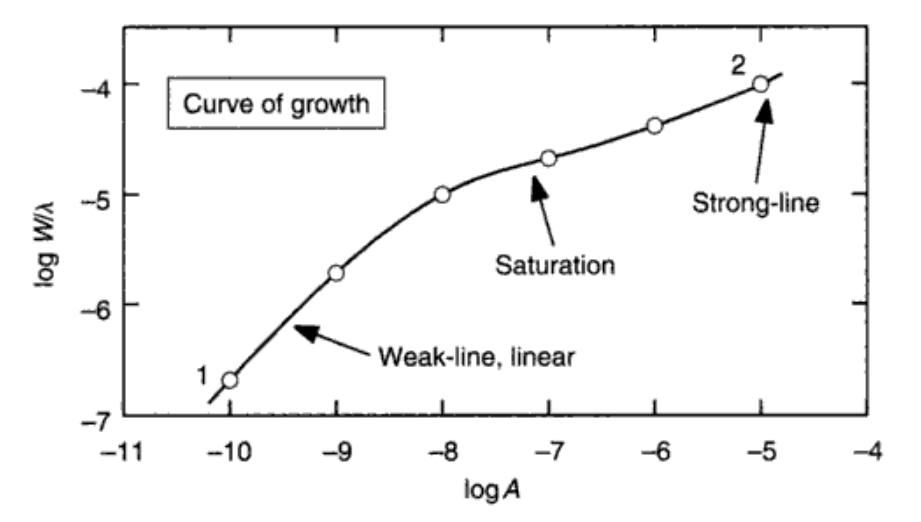# OASP笔记 - 13

## 谱线的行为

Posted by mingjie on October 7, 2018

Image credit: Jonathan Walker.

### 谱线的转移方程

$l_\nu$为谱线吸收系数，$j^l_\nu$为谱线发射系数；对于连续谱这两个量是$\kappa_\nu, j^c_\nu$。那么对于式子$(7.1)$，我们还有同样的形式：

### 谱线的源函数$(6.8)$拿过来消掉所有的AABB：

[2018.9] LTE的东西当然比较好用，不过最近在华沙的一个Gaia Workshop里面Maria Bergermann把LTE批判了一番，说nLTE对于元素丰度确定来说很重要。
[2019.6] 天可怜见我现在也开始搞nLTE啦….

### LTE下的谱线轮廓计算

No more nLTE things. 究竟什么时候LTE是好的得通过实际比较才能知道，或者问Maria去。

$(13.7)$中的$\tau_\nu = (4\pi-2)/3 = \tau_1$，有

$\tau_c,\tau_\nu$指的是没有/有谱线下的光深，并且LTE下谱线以及连续谱的源函数都是黑体辐射函数。

（不熟悉的话参见第九章$(9.13)$后面的式子）

$S_\nu(\tau_\nu=\tau_1) = S_\nu(\tau_l+\tau_c=\tau_1) = S_\nu(\tau_c=\tau_1-\tau_l)$以及$\tau_l \ll \tau_c$，泰勒展开有：

$\tau_l = \frac{l_\nu}{\kappa_0}\tau_0$代入，有

### 谱线的贡献函数

“王老五就是未结婚的王老五”，贡献函数就是第九章的贡献函数。不多说了，放两张图自己体会。### 谱线强度的行为

#### 温度

Case 1时，是原子谱线+中性原子：

#### 金属丰度

$N$为吸收原子个数，$N_E$为这种原子的总个数。

$\tau_\nu \ll 1$，即谱线很弱的时候，我们有### 最后的碎碎念

LTE和nLTE都有可能出错